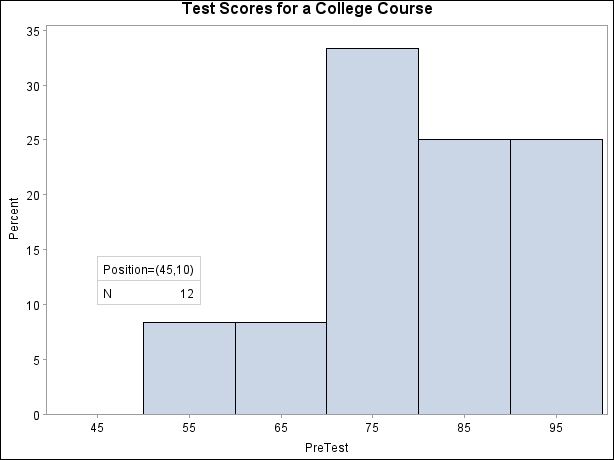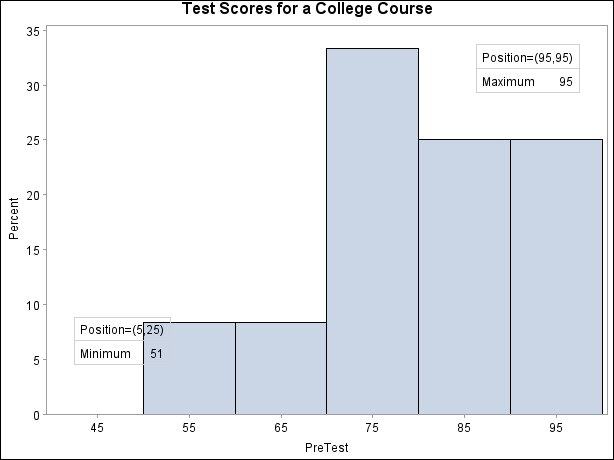# The UNIVARIATE Procedure

### Positioning Insets

Subsections:

#### Positioning an Inset Using Compass Point Values

To position an inset by using a compass point position, specify the value N, NE, E, SE, S, SW, W, or NW with the POSITION= option. The default position of the inset is NW. The following statements produce a histogram to show the position of the inset for the eight compass points:

```data Score;
input Student \$ PreTest PostTest @@;
label ScoreChange = 'Change in Test Scores';
ScoreChange = PostTest - PreTest;
datalines;
Capalleti  94 91  Dubose     51 65
Engles     95 97  Grant      63 75
Krupski    80 75  Lundsford  92 55
Mcbane     75 78  Mullen     89 82
Nguyen     79 76  Patel      71 77
Si         75 70  Tanaka     87 73
;
```
```title 'Test Scores for a College Course';
ods graphics off;
proc univariate data=Score noprint;
histogram PreTest / midpoints = 45 to 95 by 10;
inset n     / cfill=blank
inset mean  / cfill=blank
header='Position = N ' pos=n ;
inset sum   / cfill=blank
inset max   / cfill=blank
header='Position = E ' pos=e ;
inset min   / cfill=blank
inset nobs  / cfill=blank
header='Position = S ' pos=s ;
inset range / cfill=blank
inset mode  / cfill=blank
header='Position = W ' pos=w ;
label PreTest = 'Pretest Score';
run;
```

Figure 4.11: Compass Positions for Inset#### Positioning Insets in the Margins

To position an inset in one of the four margins that surround the plot area, specify the value LM, RM, TM, or BM with the POSITION= option. Margin positions are recommended if you list a large number of statistics in the INSET statement. If you attempt to display a lengthy inset in the interior of the plot, the inset is likely to collide with the data display.

#### Positioning an Inset Using Coordinates

To position an inset with coordinates, use POSITION=(x,y). You specify the coordinates in axis data units or in axis percentage units (the default). Note: You cannot position an inset with coordinates when producing ODS Graphics output.

If you specify the DATA option immediately following the coordinates, PROC UNIVARIATE positions the inset by using axis data units. For example, the following statements place the bottom left corner of the inset at 45 on the horizontal axis and 10 on the vertical axis:

```title 'Test Scores for a College Course';
proc univariate data=Score noprint;
histogram PreTest / midpoints = 45 to 95 by 10;
inset n / header   = 'Position=(45,10)'
position = (45,10) data;
run;
```

Figure 4.12: Coordinate Position for InsetBy default, the specified coordinates determine the position of the bottom left corner of the inset. To change this reference point, use the REFPOINT= option (see below).

If you omit the DATA option, PROC UNIVARIATE positions the inset by using axis percentage units. The coordinates in axis percentage units must be between 0 and 100. The coordinates of the bottom left corner of the display are (0,0), while the upper right corner is (100, 100). For example, the following statements create a histogram and use coordinates in axis percentage units to position the two insets:

```title 'Test Scores for a College Course';
proc univariate data=Score noprint;
histogram PreTest / midpoints = 45 to 95 by 10;
inset min / position = (5,25)
refpoint = tl;
inset max / position = (95,95)